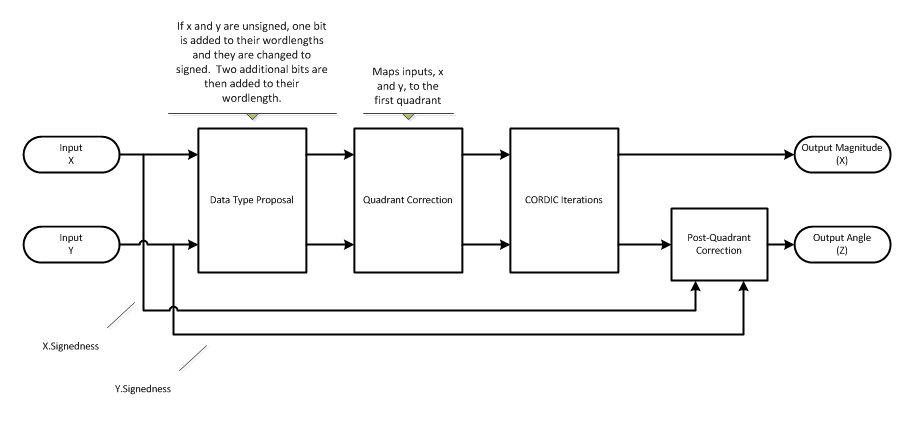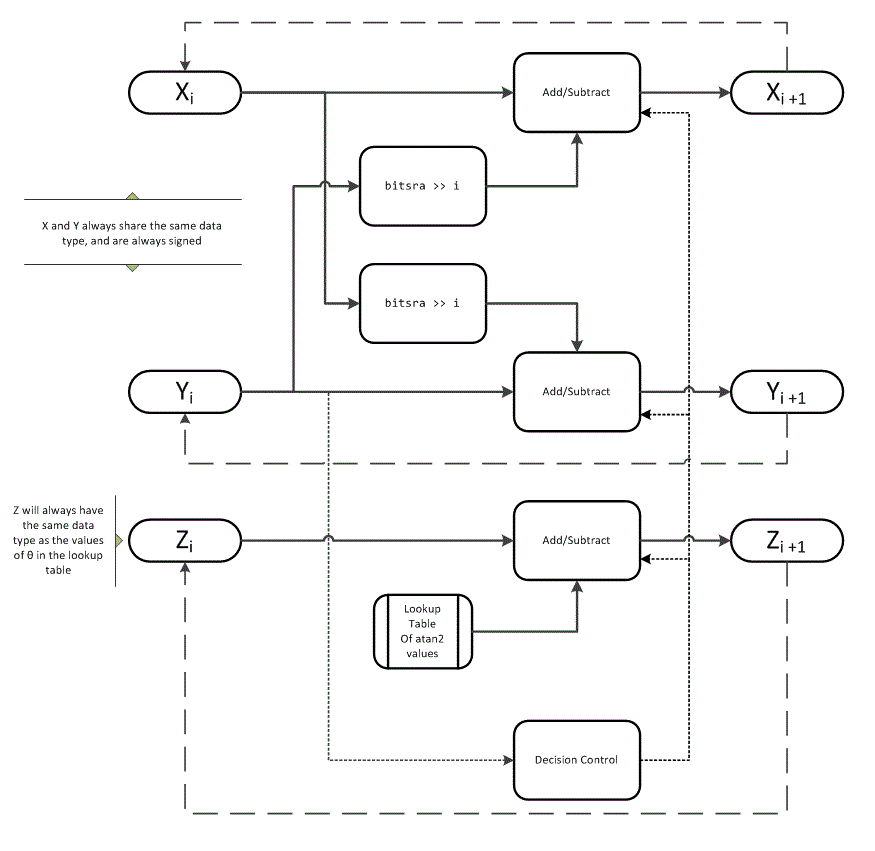Main Content

# cordiccart2pol

CORDIC-based approximation of Cartesian-to-polar conversion

## Syntax

```[theta,r] = cordiccart2pol(x,y) [theta,r] = cordiccart2pol(x,y, niters) [theta,r] = cordiccart2pol(x,y, niters,'ScaleOutput',b) [theta,r] = cordiccart2pol(x,y, 'ScaleOutput',b) ```

## Description

`[theta,r] = cordiccart2pol(x,y)` using a CORDIC algorithm approximation, returns the polar coordinates, angle `theta` and radius `r`, of the Cartesian coordinates, `x` and `y`.

`[theta,r] = cordiccart2pol(x,y, niters)` performs `niters` iterations of the algorithm.

`[theta,r] = cordiccart2pol(x,y, niters,'ScaleOutput',b)` specifies both the number of iterations and, depending on the Boolean value of `b`, whether to scale the `r` output by the inverse CORDIC gain value.

```[theta,r] = cordiccart2pol(x,y, 'ScaleOutput',b)``` scales the `r` output by the inverse CORDIC gain value, depending on the Boolean value of `b`.

## Input Arguments

 `x,y` `x,y` are Cartesian coordinates. `x` and `y` must be the same size. If they are not the same size, at least one value must be a scalar value. Both `x` and `y` must have the same data type. `niters` `niters` is the number of iterations the CORDIC algorithm performs. This argument is optional. When specified, `niters` must be a positive, integer-valued scalar. If you do not specify `niters`, or if you specify a value that is too large, the algorithm uses a maximum value. For fixed-point operation, the maximum number of iterations is the word length of `r` or one less than the word length of `theta`, whichever is smaller. For floating-point operation, the maximum value is 52 for double or 23 for single. Increasing the number of iterations can produce more accurate results but also increases the expense of the computation and adds latency.

### Name-Value Pair Arguments

Optional comma-separated pairs of `Name,Value` arguments, where `Name` is the argument name and `Value` is the corresponding value. `Name` must appear inside single quotes (`''`).

 `'ScaleOutput'` `ScaleOutput` is a Boolean value that specifies whether to scale the output by the inverse CORDIC gain factor. This argument is optional. If you set `ScaleOutput` to `true` or `1`, the output values are multiplied by a constant, which incurs extra computations. If you set `ScaleOutput` to `false` or `0`, the output is not scaled. Default: `true`

## Output Arguments

 `theta` `theta` contains the polar coordinates angle values, which are in the range [–pi, pi] radians. If `x` and `y` are floating-point, then `theta` has the same data type as `x` and `y`. Otherwise, `theta` is a fixed-point data type with the same word length as `x` and `y` and with a best-precision fraction length for the [-pi, pi] range. `r` `r` contains the polar coordinates radius magnitude values. `r` is real-valued and can be a scalar value or have the same dimensions as `theta` If the inputs `x,y` are fixed-point values, `r` is also fixed point (and is always signed, with binary point scaling). Both `x,y` input values must have the same data type. If the inputs are signed, then the word length of `r` is the input word length + 2. If the inputs are unsigned, then the word length of `r` is the input word length + 3. The fraction length of `r` is always the same as the fraction length of the `x,y` inputs.

## Examples

Convert fixed-point Cartesian coordinates to polar coordinates.

```[thPos,r]=cordiccart2pol(sfi([0.75:-0.25:-1.0],16,15),sfi(0.5,16,15)) thPos = 0.5881 0.7854 1.1072 1.5708 2.0344 2.3562 2.5535 2.6780 DataTypeMode: Fixed-point: binary point scaling Signedness: Signed WordLength: 16 FractionLength: 13 r = 0.9014 0.7071 0.5591 0.5000 0.5591 0.7071 0.9014 1.1180 DataTypeMode: Fixed-point: binary point scaling Signedness: Signed WordLength: 18 FractionLength: 15 [thNeg,r]=... cordiccart2pol(sfi([0.75:-0.25:-1.0],16,15),sfi(-0.5,16,15)) thNeg = -0.5881 -0.7854 -1.1072 -1.5708 -2.0344 -2.3562 -2.5535 -2.6780 DataTypeMode: Fixed-point: binary point scaling Signedness: Signed WordLength: 16 FractionLength: 13 r = 0.9014 0.7071 0.5591 0.5000 0.5591 0.7071 0.9014 1.1180 DataTypeMode: Fixed-point: binary point scaling Signedness: Signed WordLength: 18 FractionLength: 15 ```

## More About

collapse all

### CORDIC

CORDIC is an acronym for COordinate Rotation DIgital Computer. The Givens rotation-based CORDIC algorithm is one of the most hardware-efficient algorithms available because it requires only iterative shift-add operations (see References). The CORDIC algorithm eliminates the need for explicit multipliers. Using CORDIC, you can calculate various functions such as sine, cosine, arc sine, arc cosine, arc tangent, and vector magnitude. You can also use this algorithm for divide, square root, hyperbolic, and logarithmic functions.

Increasing the number of CORDIC iterations can produce more accurate results, but doing so increases the expense of the computation and adds latency.

## Algorithms

collapse all

### Signal Flow DiagramsCORDIC Vectoring KernelThe accuracy of the CORDIC kernel depends on the choice of initial values for X, Y, and Z. This algorithm uses the following initial values:

### fimath Propagation Rules

CORDIC functions discard any local `fimath` attached to the input.

The CORDIC functions use their own internal `fimath` when performing calculations:

• `OverflowAction``Wrap`

• `RoundingMethod``Floor`

The output has no attached `fimath`.

## See Also

Introduced in R2011b

## SupportGet trial now